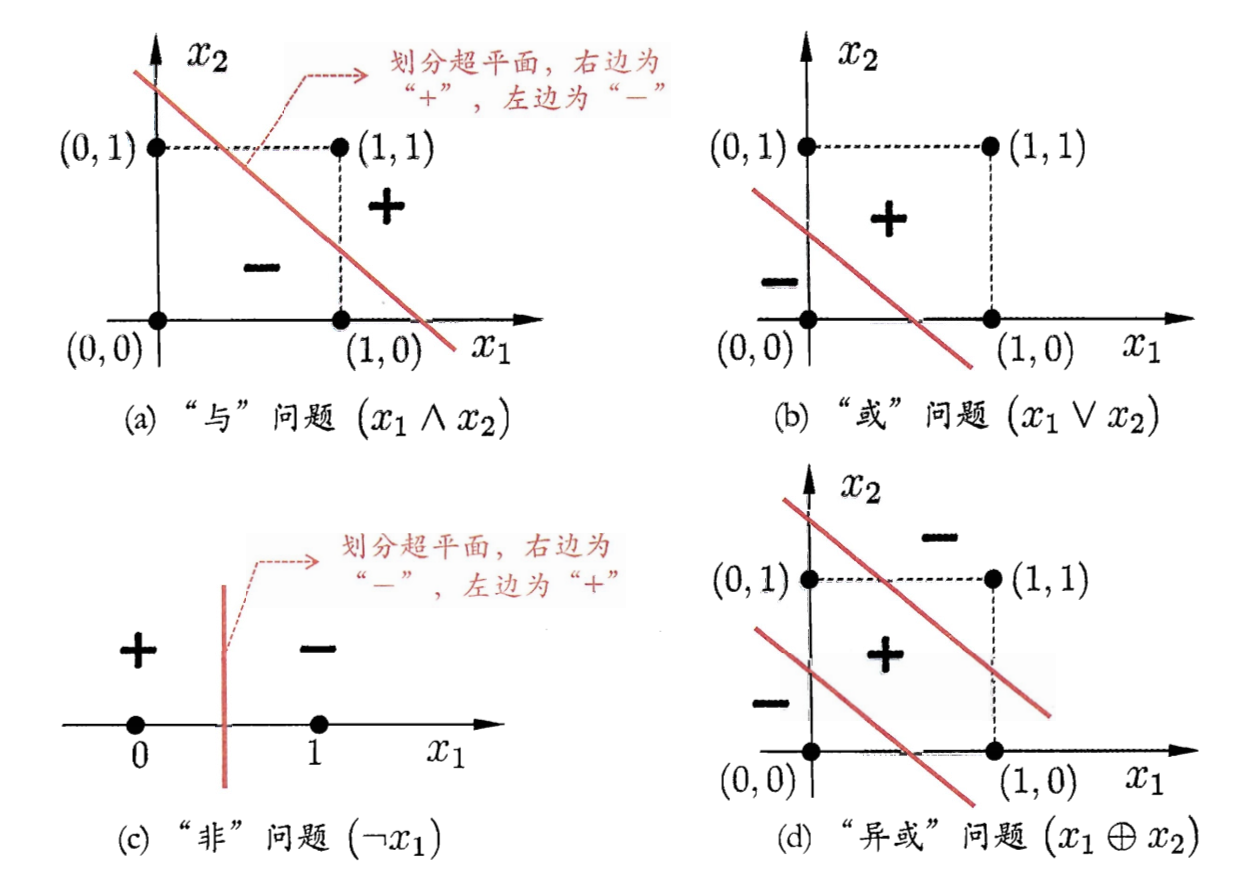# 深度学习：多层感知机和异或问题(Pytorch实现)## 感知机模型

\begin{aligned} f(x)= g(\textbf{w}\cdot \textbf{x} b) \end{aligned} \tag{1}

$$b \in \textbf{R}$$叫做偏置(bias)，$$\textbf{w}\cdot \textbf{x}$$叫做$$\textbf{w}$$$$\textbf{x}$$的内积。$$g$$是表示激活函数。理想中的中的激活函数是“阶跃函数”，即

\begin{aligned} \mathrm{sgn}(x)=\left\{ \begin{aligned} 1, \quad x \geqslant 0\\ 0, \quad x<0 \end{aligned} \right . \end{aligned} \tag{2}

，因而实际常用下面这个$$\text{Sigmoid}$$函数做为激活函数，它把可能在较大范围内变化的输入值挤压到$$(0,1)$$输出值范围内，因而有时也被称为“挤压函数”(squashing function)。

\begin{aligned} \mathrm{sigmoid}(x)=\frac{1}{1 e^{-x}} \end{aligned}

## 异或问题

import numpy as np
import random
import torch
# batch_size表示单批次用于参数估计的样本个数
# y_pred大小为(batch_size, )
# y大小为(batch_size, )，为类别型变量
def log_loss(y_pred, y):
return -(torch.mul(y, torch.log(y_pred))   torch.mul(1-y, torch.log(1-y_pred))).sum()/y_pred.shape

# 前向函数
def perceptron_f(X, w, b):
z = torch.add(torch.matmul(X, w), b)
return 1/(1 torch.exp(-z))

# 之前实现的梯度下降法，做了一些小修改
def gradient_descent(X, w, b, y, n_iter, eta, loss_func, f):
for i in range(1, n_iter 1):
y_pred = f(X, w, b)
loss_v = loss_func(y_pred, y)
loss_v.backward()
w_star = w.detach()
b_star = b.detach()
return w_star, b_star

# 本模型按照二分类架构设计
def Perceptron(X, y, n_iter=200, hidden_size=2, eta=0.001, loss_func=log_loss, optimizer=gradient_descent):
# 初始化模型参数
# 注意，各权重初始化不能相同
w = torch.tensor(np.random.random((hidden_size, )), requires_grad=True)
b = torch.tensor(np.random.random((1)), requires_grad=True)
X, y = torch.tensor(X), torch.tensor(y)
# 调用梯度下降法对函数进行优化
# 这里采用单次迭代对所有样本进行估计，后面我们会介绍小批量法
w_star, b_star = optimizer(X, w, b, y, n_iter, eta, log_loss, perceptron_f)
return w_star, b_star

if __name__ == '__main__':
X = np.array([
[0, 0],
[0, 1],
[1, 0],
[1, 1]
], dtype=np.float64)
# 标签向量
y = np.array([0, 1, 1, 0], dtype=np.int64)
# 迭代次数
n_iter = 2000
# 学习率
eta = 2 #因为每轮所求的梯度太小，这里增大学习率以补偿
w, b = Perceptron(X, y, n_iter, hidden_size, eta, log_loss, gradient_descent)
# 代入
print(perceptron_f(torch.tensor(X), w, b))


    tensor([0.5000, 0.5000, 0.5000, 0.5000], dtype=torch.float64)## 多层感知机

$$f^{(1)}$$应该采用那种函数？如果我们仍然采用线性函数，那么前馈网络作为一个整体仍然是线性分类器。故我们要用非线性函数，而这可以通过仿射变换后加一个非线性变换实现
（不知道仿射变换的可以回顾线性代数），而这个非线性变换可以用我们在$$f^{(2)}$$中所包括的激活函数实现。

import numpy as np
import random
import torch
# batch_size表示单批次用于参数估计的样本个数
# y_pred大小为(batch_size, )
# y大小为(batch_size, )，为类别型变量
def log_loss(y_pred, y):
return -(torch.mul(y, torch.log(y_pred))   torch.mul(1-y, torch.log(1-y_pred))).sum()/y_pred.shape

# 前向函数
def perceptron_f(X, W, w, b1, b2):
z1 = torch.add(torch.matmul(X, W), b1)
h = 1/(1 torch.exp(-z1))
z2 = torch.add(torch.matmul(h, w), b2)
return 1/(1 torch.exp(-z2))

# 之前实现的梯度下降法，做了一些小修改
def gradient_descent(X, W, b1, w, b2, y, n_iter, eta, loss_func, f):
for i in range(1, n_iter 1):
y_pred = f(X, W, w, b1, b2)
loss_v = loss_func(y_pred, y)
loss_v.backward()
W_star = W.detach()
w_star = w.detach()
b1_star = b1.detach()
b2_star = b2.detach()
return W_star, w_star, b1_star, b2_star

# 本模型按照二分类架构设计
def Perceptron(X, y, n_iter=200, hidden_size=2, eta=0.001, loss_func=log_loss, optimizer=gradient_descent):
# 初始化模型参数
# 注意，各权重初始化不能相同
W = torch.tensor(np.random.random((X.shape, hidden_size)), requires_grad=True)
b1 = torch.tensor(np.random.random((1)), requires_grad=True)
w = torch.tensor(np.random.random((hidden_size, )), requires_grad=True)
b2 = torch.tensor(np.random.random((1)), requires_grad=True)
X, y = torch.tensor(X), torch.tensor(y)
# 调用梯度下降法对函数进行优化
# 这里采用单次迭代对所有样本进行估计，后面我们会介绍小批量法
W_star, w_star, b1_star, b2_star = optimizer(X, W, b1, w, b2, y, n_iter, eta, log_loss, perceptron_f)
return W_star, w_star, b1_star, b2_star

if __name__ == '__main__':
X = np.array([
[0, 0],
[0, 1],
[1, 0],
[1, 1]
], dtype=np.float64)
# 标签向量
y = np.array([0, 1, 1, 0], dtype=np.int64)
# 迭代次数
n_iter = 2000
# 学习率
eta = 2 #因为每轮所求的梯度太小，这里增大学习率以补偿
# 隐藏层神经元个数
hidden_size = 2
W, w, b1, b2 = Perceptron(X, y, n_iter, hidden_size, eta, log_loss, gradient_descent)
# 代入
print(perceptron_f(torch.tensor(X), W, w, b1, b2))



    tensor([0.0036, 0.9973, 0.9973, 0.0030], dtype=torch.float64)


0条回复